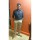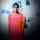Java Developers
Group for Java developers and programmers. Ask questions and get answers related to Java programming
255 Members
Join this group to post and comment.Computer Science
15 Jan 2019

# What is the difference between equals() and == ?

Give examples to support it?Puspanjali Jena
Computer Science
2mos ago

==  operator                                                        __________

1.we can use it for comparing two primivitive values,objects,and nulls.

2. It always compares object with references.

3.we cannot compare incompatible  objects, then compiler throws error.

Equals() method

_____________

1.we can use it only for comparing two objects. It cannot be used for comparing primitive values and nulls it leads to comiple time error becoz a method cannot be invoking by using primitive values and nulls.

2. It compares  object either with reference or with state based on its implementation.

3.we can compares incompatible objects using equals() method it returns  false.DHIRAJ LOTLIKAR
Information Technology
2mos ago

The equals method is meant to compare the contents of two objects and not their location in memory. In java the == operator is used to compare two objects locations but not its content.

For example,

public class Example{

public static void main(String[] args){

String obj1 = new String('abc');

String obj2 = new String('abc');

If(obj1 == obj2){

System.out.println('Obj1 == obj2 is true');

else

System.out.println('Obj1 == obj2 is false');

}

}

For the above example the output will be 'Obj1 == obj2 is false'. If you replace the If condition by

If(obj1.equals(obj2))

then the output will be 'Obj1 == obj2 is true'.Computer Science
2mos ago

Both equals() method and == are used  to compare the objects to check equality but there are some differences between these two:

equals() method:It is a method used to compare both primitive and objects.And compares the two given strings based on the content of the string.If all the characters are matched then it returns true and   if atleast a single character is not matched then it returns false.

For example:

class Test

{

public static void main(String s[])

String s="example";

String s1="example";

String s2="Example":

System.out.println(s.equals(s1));//returns true as both the characters and case is same

System.out.println(s1.equals(s2));//returns false because the characters are same but the case is not same the character in s2 is uppercase.

== operator:It is an operator which compares the objects based on their location in memory.Compares the reference of variables not their values.

For example:

class Test

public static void main(String s[])

{

String s="test";

String s1="test";

String s2=new String("test");

System.out.println(s==s1);

//returns true as both are referring to same object.

System.out.println(s1==s2);

//returns false as when the string is created using new operator it is created in the heap space so it returns false when comparing s1 and s2.